# Algebra 1 : Decimals and Percentage

## Example Questions

### Example Question #91 : Decimals And Percentage

Rewrite the following percent as a decimal: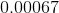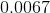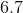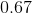Explanation:

Rewrite the following percent as a decimal: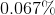To convert between decimals and percents, we simply need to move the decimal point.

When going from a decimal to a percent, move the point two spaces to the right.

When going from a percent to a decimal, move the point two spaces to the left.

So this,Becomes### Example Question #92 : Decimals And Percentage

Which of the following is the decimal equivalent of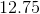%?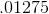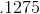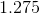Explanation:

To find the decimal equivalent of a percentage, simply move the decimal (if there is none, remember that there is always an implied decimal after the one's digit) to the left twice.  The best answer is:### Example Question #92 : Decimals And Percentage

Which of the following is the decimal equivalent of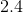%?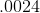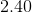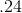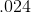Explanation:

To find the decimal equivalent of a percentage, simply move the decimal (if there is none, remember that there is always an implied decimal after the one's digit) to the left twice.  The best answer is:### Example Question #93 : Decimals And Percentage

Which of the following is the decimal equivalent of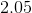%?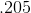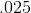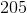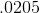Explanation:

To find the decimal equivalent of a percentage, simply move the decimal (if there is none, remember that there is always an implied decimal after the one's digit) to the left twice.  The best answer is:### Example Question #95 : Decimals And Percentage

Which of the following is the decimal equivalent of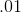%?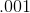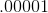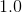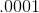Explanation:

To find the decimal equivalent of a percentage, simply move the decimal (if there is none, remember that there is always an implied decimal after the one's digit) to the left twice.  The best answer is:### Example Question #51 : How To Find Decimal Equivalent To A Percentage

Find the percent equivalent of .043.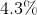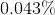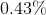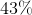Explanation:

To solve, simply remember that to convert from a decimal to a percent, you simply have to multiply it by 100.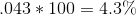If multiplying by 100 isn't easily memorizable for you, simply move the decimal place to spots to the right. You get the same answer!

### Example Question #51 : How To Find Decimal Equivalent To A Percentage

Find the decimal given the following percentage: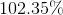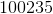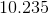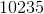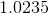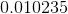Explanation:

In order to find the decimal for a percentage, simply remove the percentage sign and divide by 100, or shift the decimal place back two spaces.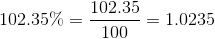The answer is:### Example Question #94 : Decimals And Percentage

Find the decimal equivalent of 37%.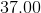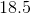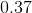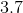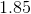Explanation:

When finding the decimal equivalent of a percent, you do the following: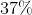is the same as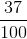So, we take 37 divided by 100, and we getSo, the decimal equivalent ofis.

### Example Question #95 : Decimals And Percentage

Find the decimal equivalent of 24.5%.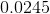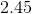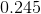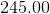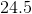Explanation:

When finding the decimal equivalent of a percent, you do the following: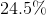is the same as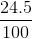So, we take 24.5 divided by 100, and we getSo, the decimal equivalent ofis.

### Example Question #96 : Decimals And Percentage

Convert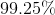into a fraction in lowest terms.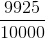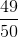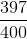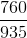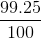Explanation:

Since this percentage includes a decimal already, this question requires changing the percentage to a decimal first. One way to do it is to divide the number by 100 (note that this is the same thing as turning the number into a fraction over 100).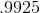Now, it helps if you know decimal place values. To turn this into a fraction, put 9925 over the place value of the furthest right digit, in this case the 5. This is the ten thousandths place so put 9925 over 10000Now reduce it to lowest terms by dividing the numerator and denominator evenly by the same number. I can see that 25 is a common factor so I will try that first.Since no number will go into both 397 and 400, this is the answer reduced to lowest terms.

### All Algebra 1 Resources